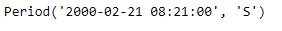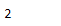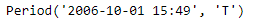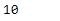Python | Pandas Period.month

• Last Updated : 06 Jan, 2019

Python is a great language for doing data analysis, primarily because of the fantastic ecosystem of data-centric python packages. Pandas is one of those packages and makes importing and analyzing data much easier.

Pandas Period.month attribute return an integer value. The returned value represents the value of month in the given period object. January being 1 to December being 12.

Syntax : Period.month

Parameters : None

Return : month

Example #1: Use Period.month attribute to find the value of month in the given Period object.

 # importing pandas as pdimport pandas as pd  # Create the Period objectprd = pd.Period(freq ='S', year = 2000, month = 2,                 day = 21, hour = 8, minute = 21)  # Print the Period objectprint(prd)

Output :Now we will use the Period.month attribute to find out value of month in prd object.

 # return value of monthprd.month

Output :As we can see in the output, the Period.month attribute has returned 2 indicating that the given period object has ‘February’ as a month.

Example #2: Use Period.month attribute to find the value of month in the given Period object.

 # importing pandas as pdimport pandas as pd  # Create the Period objectprd = pd.Period(freq ='T', year = 2006, month = 10, hour = 15, minute = 49)  # Print the Period objectprint(prd)

Output :Now we will use the Period.month attribute to find out value of month in prd object.

 # return value of monthprd.month

Output :As we can see in the output, the Period.month attribute has returned 10 indicating that the given period object has ‘October’ as a month.

My Personal Notes arrow_drop_up